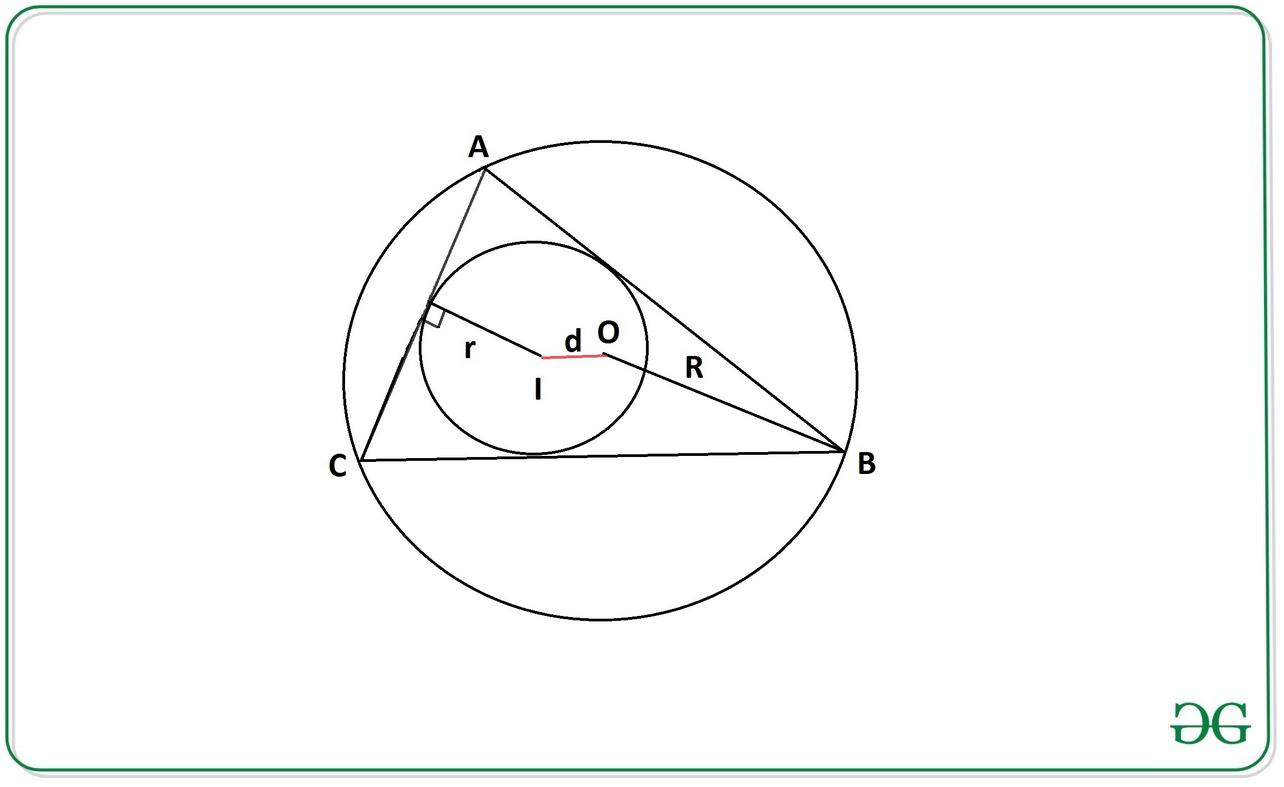Open in App
Not now

# Distance between Incenter and Circumcenter of a triangle using Inradius and Circumradius

• Difficulty Level : Easy
• Last Updated : 09 Sep, 2022

Given two integers r and R representing the length of Inradius and Circumradius respectively, the task is to calculate the distance d between Incenter and Circumcenter.Inradius The inradius( r ) of a regular triangle( ABC ) is the radius of the incircle (having center as l), which is the largest circle that will fit inside the triangle.
Circumradius: The circumradius( R ) of a triangle is the radius of the circumscribed circle (having center as O) of that triangle.

Examples:

Input: r = 2, R = 5
Output: 2.24

Input: r = 5, R = 12
Output: 4.9

Approach:
The problem can be solved using Euler’s Theorem in geometry, which states that the distance between the incenter and circumcenter of a triangle can be calculated by the equation:Below is the implementation of the above approach:

## C++14

 // C++14 program for the above approach #include  using namespace std;   // Function returns the required distance double distance(int r, int R)  {      double d = sqrt(pow(R, 2) -                         (2 * r * R));                                    return d;  }    // Driver code  int main()  {            // Length of Inradius      int r = 2;            // Length of Circumradius      int R = 5;        cout << (round(distance(r, R) * 100.0) / 100.0);  }    // This code is contributed by sanjoy_62

## Java

 // Java program for the above approach  import java.util.*;   class GFG{       // Function returns the required distance static double distance(int r,int R) {     double d = Math.sqrt(Math.pow(R, 2) -                          (2 * r * R));                                return d; }   // Driver code public static void main(String[] args) {           // Length of Inradius     int r = 2;           // Length of Circumradius     int R = 5;       System.out.println(Math.round(         distance(r, R) * 100.0) / 100.0); } }   // This code is contributed by offbeat

## Python3

 # Python3 program for the above approach import math   # Function returns the required distance def distance(r,R):       d = math.sqrt( (R**2) - (2 * r * R))           return d   # Driver Code   # Length of Inradius r = 2   # Length of Circumradius R = 5   print(round(distance(r,R),2))

## C#

 // C# program for the above approach  using System;   class GFG{       // Function returns the required distance static double distance(int r, int R) {     double d = Math.Sqrt(Math.Pow(R, 2) -                          (2 * r * R));                               return d; }   // Driver code public static void Main(string[] args) {           // Length of Inradius     int r = 2;           // Length of Circumradius     int R = 5;           Console.Write(Math.Round(         distance(r, R) * 100.0) / 100.0); } }   // This code is contributed by rutvik_56

## Javascript

 

Output:

2.24

Time Complexity: O(logn) since time complexity of sqrt is O(logn)
Auxiliary Space: O(1)

My Personal Notes arrow_drop_up
Related Articles